Home | | Physics 12th Std | Solved Example Numerical Problems

# Solved Example Numerical Problems

Physics : Wave Optics: Book Back, Exercise, Example Numerical Question with Answers, Solution: Solved Example Numerical Problems

Wave Optics - Numerical Problems Questions with Answers, Solution

Wave Nature of Light - Numerical Problems Questions with Answers, Solution

### EXAMPLE Q

The wavelength of light from sodium source in vacuum is 5893Å.What are its (a) wavelength, (b) speed and (c) frequency when this light travels in water which has a refractive index of 1.33.

### Solution

The refractive index of vacuum, n1 = 1

The wavelength in vacuum, λ1 = 5893 Å.

The speed in vacuum, = 3 × 108 m s–1

The refractive index of water, n2 = 1.33

The wavelength of light in water, λ2

The speed of light in water, v2

(a) The equation relating the wavelength and refractive index is,(b) The equation relating the speed and refractive index is,The results show that the frequency remains same in all media.

Interference - Numerical Problems Questions with Answers, Solution

EXAMPLE Q

Two light sources with amplitudes 5 units and 3 units respectively interfere with each other. Calculate the ratio of maximum and minimum intensities.

Solution

Amplitudes, a1 = 5, a2 = 3

Resultant amplitude,EXAMPLE Q

Two light sources of equal amplitudes interfere with each other. Calculate the ratio of maximum and minimum intensities.

Solution

Let the amplitude be a.

The intensity is,  µ 4a2 cos2(ϕ/ 2)

or = 4I0 cos2(ϕ/2)

Resultant intensity is maximum when,

ϕ  = 0, cos0 = 1, Imax  4a2

Resultant amplitude is minimum when,

ϕ = π, cos(π/2) = 0, Imin = 0

Imax : Imin = 4a2 : 0

EXAMPLE Q

Two light sources have intensity of light as I0. What is the resultant intensity at a point where the two light waves have a phase difference of π/3?

Solution

Let the intensities be I0.

The resultant intensity is, = 4I0cos2(ϕ/2)

Resultant intensity when, ϕ π / 3, is

I = 4I0 cos2 (π/6)

I = 4Io (√3/2)2 = 3Io

EXAMPLE Q

The wavelength of a light is 450 nm. How much phase it will differ for a path of 3 mm?

Solution

The wavelength is, λ = 450 nm = 450×10-9m

Path difference is, δ = 3 mm = 3×10−3m

Relation between phase difference and path difference is, ϕ = 2π/λ ×δ

Substituting,

ϕ = [ 2π / 450×10-9 ] ×3×10−3 = (π/75) ×106

### EXAMPLE Q

In Young’s double slit experiment, the two slits are 0.15 mm apart. The light source has a wavelength of 450 nm. The screen is 2 m away from the slits.

(i) Find the distance of the second bright fringe and also third dark fringe from the central maximum.

(ii) Find the fringe width.

(iii) How will the fringe pattern change if the screen is moved away from the slits?

(iv) What will happen to the fringe width if the whole setup is immersed in water of refractive index 4/3.

Solution

= 0.15 mm = 0.15× 10-3 m; = 2 m;

λ = 450 nm = 450 × 10-9 m; = 4/3

(i) Equation for nth bright fringe is,(ii) Equation for fringe width is,(iii) The fringe width will increase as D is increased,(iv) The fringe width will decrease as the setup is immersed in water of refractive index 4/3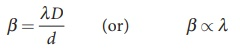The wavelength will decrease refractive index times.

Hence, β  λ and β’  λ’### EXAMPLE Q

Two lights of wavelengths 560 nm and 420 nm are used in Young’s double slit experiment. Find the least distance from the central fringe where the bright fringe of the two wavelengths coincides. Given = 1 m and d = 3 mm.

### Solution

λ = 560 nm = 560×10−9 m;

λ2 = 420 nm = 420×10−9 m;

= 1 m;= 3 mm = 3×10−3 m

For a given yand λ are inversely proportional.

Let nth order bright fringe of λ1 coincides with (n+1)th order bright fringe of λ2.Thus, the 3rd bright fringe of λ1 and 4th bright fringe of λ2 coincide at the least distance y.

The least distance from the central fringe where the bright fringes of the two wavelengths coincides is, yn [(λD)/d]### EXAMPLE Q

Find the minimum thickness of a film of refractive index 1.25, which will strongly reflect the light of wavelength 589 nm. Also find the minimum thickness of the film to be anti-reflecting.

### Solution

λ = 589 nm = 589×10−9 m

For the film to have strong reflection, the reflected waves should interfere constructively. The least optical path difference introduced by the film should be λ/2. The optical path difference between the waves reflected from the two surfaces of the film is 2µd. Thus, for strong reflection, 2µd λ/2 [As given in equation 6.145. with = 1]

Rewriting, = λ/4μ

Substituting, = [589×109] / [4×1.25] = 117.8×10−9

= 117.8×10−9 = 117.8 nm

For the film to be anti-reflecting, the reflected rays should interfere destructively. The least optical path difference introduced by the film should be λ. The optical path difference between the waves reflected from the two surfaces of the film is 2µd. For strong reflection, 2µd λ [As given in equation 6.146. with = 1].

Rewriting, = λ/2μ

Substituting, = [589×109] / [2×1.25] = 235.6×10−9

= 235.6×10−9 = 235.6 nm

### Diffraction - Numerical Problems Questions with Answers, Solution

EXAMPLE Q

Light of wavelength 500 nm passes through a slit of 0.2 mm wide. The diffraction pattern is formed on a screen 60 cm away. Determine the,

(i) angular spread of central maximum

(ii) the distance between the central maximum and the second minimum.

Solution

λ = 500 nm = 500×10-9 m; = 0.2 mm = 0.2×10-3 m; = 60 cm = 60×10-2 m

(i) Equation for diffraction minimum is, sin θ nλ

The central maximum is spread up to the first minimum. Hence, = 1(ii) To find the value of y1 for central maximum, which is spread up to first minimum with (= 1) is, sin θ λ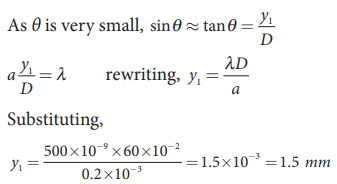To find the value of y2 for second minimum with (n = 2) is, a sin θ = 2λThe distance between the central maximum and second minimum is, y2 – y1

y2 – y1 = 3 mm – 1.5 mm = 1.5 mmNote: The above calculation shows that in the diffraction pattern caused by single slit, the width of each maximum is equal with central maximum as the double that of others. But the bright and dark fringes are not of equal width.

EXAMPLE Q

A monochromatic light of wavelength 5000 Å passes through a single slit producing diffraction pattern for the central maximum as shown in the figure. Determine the width of the slit.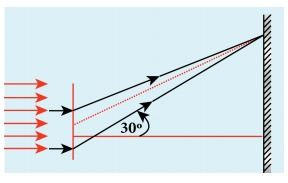Solution

λ = 5000 Å = 5000×10-10 m; sin 30º = 0.5; = 1; =?

Equation for diffraction minimum is, asin θ

The central maximum is spread up to the first minimum. Hence, = 1

Rewriting, = λ / Sinθ

Substituting, = 5000×10−10 / 0.5

= 1×10−6 = 0.001×10−3= 0.001mm

EXAMPLE Q

Calculate the distance for which ray optics is good approximation for an aperture of 5 mm and wavelength 500 nm.

Solution

= 5 mm = 5 × 10-3 m;

λ = 500nm = 500×10−9 m= ?

Equation for Fresnel’s distance, a2/2λ

Substituting,

= [5 × 10-3]/ 2×500×10−9

= 25 m

### EXAMPLE Q

A diffraction grating consisting of 4000 slits per centimeter is illuminated with a monochromatic light that produces the second order diffraction at an angle of 30°. What is the wavelength of the light used?

### Solution

Number of lines per cm = 4000; = 2;

θ = 30°; λ = ?

Number of lines per unit length,

= 4000 / 1×10−2 = 4×105

Equation for diffraction maximum in grating is, sinθ Nmλ

Rewriting, λ = sinθ / Nm

Substituting,

λ = sin 30º / 4×105×2 =  0.5 / 4×105×2

= 1 / [2×4×105 ×2] = 1 / [16×105]

λ = 6250×10−10 = 6250 Ao

### EXAMPLE Q

A monochromatic light of wavelength of 500 nm strikes a grating and produces fourth order bright line at an angle of 30°. Find the number of slits per centimeter.

### Solution

λ = 500 nm = 500×10-9 m; = 4;

θ = 30°; number of lines per cm = ?

Equation for diffraction maximum in grating is, sin θ Nm λ

Rewriting, = sinθ / mλ

Substituting,

N = 0.5 / 4×500×10−9 = 1 / 2×4×500×10−9

N = 2.5×105 lines per meter

number of lines per centimeter = 2.5×105 ×10−2 = 2500 lines per centimetre

### EXAMPLE Q

The optical telescope in the Vainu Bappu observatory at Kavalur has an objective lens of diameter 2.3 m. What is its angular resolution if the wavelength of light used is 589 nm?

### Solution

a = 2.3 m; λ = 589 nm = 589×10-9 m; θ = ?

The equation for angular resolution is,

θ = 1.22 λ  a

Substituting,

θ = 1.22×589×10−9 / 2.3 = 321.4×10−9

θ = 3.214×10−7 rad ≈ 0.0011'

Note: The angular resolution of human eye is approximately, 3×10−4 rad ≈ 1.03'.

### EXAMPLE Q

Two polaroids are kept with their transmission axes inclined at 30º. Unpolarised light of intensity falls on the first polaroid. Find out the intensity of light emerging from the second polaroid.

### Solution

As the intensity of the unpolarised light falling on the first polaroid is I, the intensity of polarized light emerging will be, I0 = ( I/2 ).

Let ‘ be the intensity of light emerging from the second polaroid.

Malus’ law, ′ = I0 cos2 θ

Substituting,### EXAMPLE Q

Two polaroids are kept crossed (transmission axes at 90º) to each other.

(i) What will be the intensity of the light coming out from the second polaroid when an unpolarised light of intensity falls on the first polaroid?

(ii) What will be the intensity of light coming out from the second polaroid if a third polaroid is kept at 45º inclination to both of them.

### Solution

(i) As the intensity of the unpolarised light falling on the first polaroid is I, the intensity of polarized light emerging from it will be I= (I/2).

Let I‘ be the intensity of light emerging from the second polaroid.

Malus’ law, ′ = I0 cos2 θ

Here θ is 90º as the transmission axes are perpendicular to each other.

Substituting,

I0 = ( I/2 ) cos2 (90º)= 0

[ cos(90º )= 0]

No light comes out from the second polaroid.

(ii) Let the first polaroid be P1 and the second polaroid be P2. They are oriented at 90º. The third polaroid P3 is introduced between them at 45º. Let ‘ be the intensity of light emerging from P3.

Angle between P1 and P3 is 45º. The intensity of light coming out from P3 is, ′ = I0 cos2 θ

Substituting,Angle between P3 and P2 is 45º. Let I” is the intensity of light coming out from P2 ′′ = ′ cos2 θ

Here, the intensity of polarized light existing between P3 and P2 is 1/4.

Substituting,### EXAMPLE Q

Find the polarizing angles for (i) glass of refractive index 1.5 and (ii) water of refractive index 1.33.

### Solution

Brewster’s law, tanip n

For glass, tanip = 1.5; ip = tan−11.5; ip = 56.3º

Forwater, tanip = 1.33; ip = tan−11.33; ip = 53.1º

### EXAMPLE Q

What is the angle at which a glass plate of refractive index 1.65 is to be kept with respect to the horizontal surface so that an unpolarised light travelling horizontal after reflection from the glass plate is found to be plane polarised?

### Solution

= 1.65

Brewster’s law, tanip n

tanip = 1.65; ip = tan−11.65; ip = 58.80

The inclination with the horizontal surface is, (900 −58.80 ) = 31.20

### EXAMPLE Q

A man with a near point of 25 cm reads a book with small print using a magnifying glass, a convex lens of focal length 5 cm. (a) What is the closest and the farthest distance at which he should keep the lens from the page so that he can read the book when viewing through the magnifying glass? (b) What is the maximum and the minimum angular magnification (magnifying power) possible using the above simple microscope?

### Solution

= 25 cm; = 5 cm;

For closest object distance, u; the image distance, is, –25 cm. (near point focusing)

For farthest object distance, u’; the corresponding image distance, v’ is, v’ = ∞ (normal focusing)

(a) To find closest image distance, lens equation, 1/v – 1/u = 1/f

Rewriting for closest object distance, 1/u = 1/v - 1/f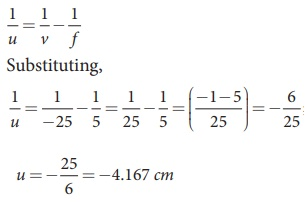The closest distance at which the person should can keep the book is, = –4.167 cm

To find farthest object distance, lens equation is, 1/v’ – 1/u’ = 1/f’

Rewriting for farthest object distance, 1/u’ = 1/v’ - 1/f’

Substituting, 1/u’ = 1/∞ - 1/5= −5 cm

The farthest distance at which the person can keep the book is, u′ = −5 cm

(b) To find magnification in near point focusing, = 1+ D/f = 1+ 25/5 = 6

To find magnification in normal focusing,

D/f = 25/5 = 5

Compound microscope - Numerical Problems Questions with Answers, Solution

### EXAMPLE Q

A microscope has an objective and eyepiece of focal lengths 5 cm and 50 cm respectively with tube length 30 cm. Find the magnification of the microscope in the (i) near point and (ii) normal focusing.

### Solution

f0 = 5cm = 5×10−2 mfe = 50cm = 50×10−2 m;

= 30cm = 30×10−2 m= 25cm = 25×10−2 m

(i) The total magnification in near point focusing is, mome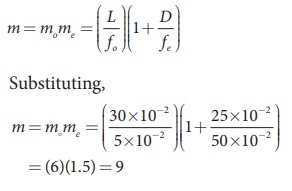(ii) The total magnification m in normal  focusing is, mome### EXAMPLE Q

A small telescope has an objective lens of focal length 125 cm and an eyepiece of focal length 2 cm. What is the magnification of the telescope? What is the separation between the objective and the eyepiece? Two stars separated by 1’ will appear at what separation when viewed through the telescope?

### Solution

fo = 125 cm; fe = 2 cm; = ?; = ?; θi = ?

Equation for magnification of telescope, fo/ fe

Substituting, = 125/2 = 62.5

Equation for approximate length of telescope, L = fo+ fe

Substituting, = 125+2 = 127 cm = 1.27 m

Equation for angular magnification, θi0

Rewriting, θi m×θ0

Substituting,

θi = 62.5×1′ = 62.5′ = 62.5/60 = 1.04º

### EXAMPLE Q

Calculate the power of the lens of the spectacles necessary to rectify the defect of nearsightedness for a person who could see clearly only up to a distance of 1.8 m.

### Solution

The maximum distance the person could see is, = 1.8 m.

The lens should have a focal length of, = –m = –1.8 m.

It is a concave or diverging lens.

The power of the lens is,

= − 1/1.8 m

= −0.56 diopter

### EXAMPLE Q

A person has farsightedness with the minimum distance he could see clearly is 75 cm. Calculate the power of the lens of the spectacles necessary to rectify the defect.

### Solution

The minimum distance the person could see clearly is, = 75 cm.

The lens should have a focal length of,= y×25 cm / y-25 cm

= [75 cm×25 cm ] / [75 cm −25 cm] = 37.5 cm

It is a convex or converging lens.

The power of the lens is,

= 1 / 0.375 m  = 2.67 diopter

Numerical Problems

1. The ratio of maximum and minimum intensities in an interference pattern is 36:1. What is the ratio of the amplitudes of the two interfering waves?

[Ans: 7:5]2. In Young’s double slit experiment, 62 fringes are seen in visible region for sodium light of wavelength 5893 Å. If violet light of wavelength 4359 Å is used in place of sodium light, then what is the number of fringes seen? [Ans: 84]3. Light of wavelength 600 nm that falls on a pair of slits producing interference pattern on a screen in which the bright fringes are separated by 7.2 mm. What must be the wavelength of another light which produces bright fringes separated by 8.1 mm with the same apparatus?

[Ans: 675 nm]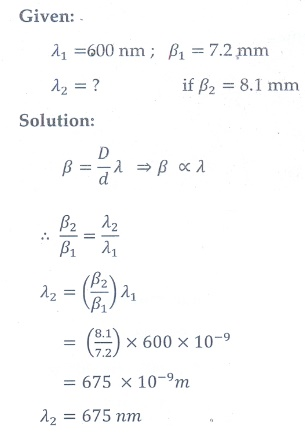4. A beam of light of wavelength 600 nm from a distant source falls on a single slit 1 mm wide and the resulting diffraction pattern is observed on a screen 2 m away. What is the distance between the first dark fringe on either side of the central bright fringe?

[Ans: 2.4 mm]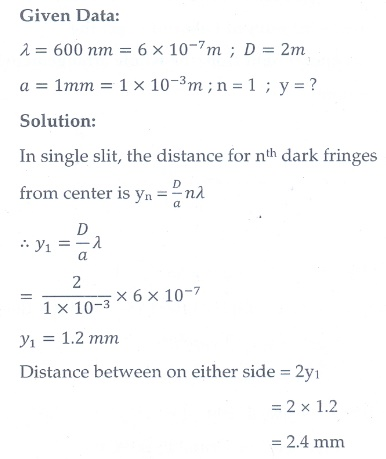5. Light of wavelength of 5000 Å produces diffraction pattern of the single slit of width 2.5 µm. What is the maximum order of diffraction possible?

[Ans: 5]6. I0 is the intensity of light existing between two cross Polaroids kept with their axes perpendicular to each other. A third polaroid is introduced between them. What must be the angle between the axes of first and the newly introduced polaroid to ge the maximum light from the whol arrangement?

[Ans: 45°]7. An unpolarised light of intensity 32 Wm-2 passes through three Polaroids such that the axes of the first and the last Polaroids are at 90°. What is the angle between the axes of the first and middle Polaroids so that the emerging light has an intensity of only 3 Wm-²?

[Ans: 30°]8. The reflected light is found to be plane polarised when an unpolarized light falls on a denser medium at 60° with the normal. Find the angle of refraction and critical angle of incidence for total internal reflection in the denser to rarer medium reflection.

[Ans: 30°, 35.15°]9. The near point and the far point for a person are 50 cm and 500 cm, respectively. Calculate the power of the lens the person should wear to read a book held in hand at 25 cm. What maximum distance is clearly visible for the person with this lens on the eye?

[Ans: 2D, 45.45 cm]10. A compound microscope has a magnifying power of 100 when the image is formed at infinity. The objective has a focal length of 0.5 cm and the tube length is 6.5 cm. What is the focal length of the eyepiece.

[Ans: 2 cm]Tags : Wave Optics | Physics , 12th Physics : UNIT 7 : Wave Optics
Study Material, Lecturing Notes, Assignment, Reference, Wiki description explanation, brief detail
12th Physics : UNIT 7 : Wave Optics : Solved Example Numerical Problems | Wave Optics | Physics